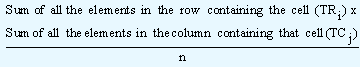## Chi square test for more than two rows, Applied Statistics

Assignment Help:

### Using Chi Square Test when more than two Rows are Present

To understand this, let us consider the contingency table shown below. It gives us the information about the stage of the economy and the number of PCs sold on a weekly basis.

 Weekly sale of PCs Total High Medium Low Economy at Peak Economy at Trough Economy Rising Economy Falling 20 13 18 14 14   12 9 10 5 9 8 44 26 39 31 Total 65 43 32 140

For calculating the proportion of sales during a weekly high, when the economy was rising we employ the formula of joint probability for two independent events A and B. The event A stands for proportion of sales durings a high irrespective of the stage of the economy and is given by (65/140), whereas the proportion of sales when the economy was rising irrespective of the weekly sales is (39/140). Since these two events happen to be independent events, the joint probability that they will occur simultaneously is

=  (65/140)(39/140)

=  2535/19600

Similarly the proportion of sales, when the weekly sales are low and the economy at its peak is given by the product of

=  (32/140)(44/140)

=  1408/19600

Since these figures give us the expected proportions to calculate the theoretical proportions, we multiply the above values with the total number of observations. Therefore, the theoretical proportion for the cell (economy rising, high) is given by

(2535/19600) x 140 = 18.107

and for the cell (economy at peak, low) the theoretical proportion is

(1408/19600) x 140 = 10.06

Generalizing this, the expected frequency for any cell (intersection point of the concerned row and the column) is given by the product of the sum of the elements in that row (usually denoted by TRi, i = 1, 2) and the sum of the elements in that column (denoted by CRj, j = 1, 2, 3) divided by the total number of observations. Mathematically it is expressed asAfter this step, we proceed in the same manner as we do in case of contingency table having two rows and a given number of columns.

#### Question with R - Bioinformatics, Hi There, I have a question regarding R,...

Hi There, I have a question regarding R, and I am wondering if anyone can help me. Here is a code that I would like to understand: squareFunc g f(x)^2 } return(g) } sin

#### Properties of standard deviation, PROPERTIES   1. The value of stand...

PROPERTIES   1. The value of standard deviation remains the same if, in a series each of the observation is increased or decreased by a constant quantity. In statistical lan

#### Calculation of degrees of freedom, Calculation of Degrees of Freedom Fi...

Calculation of Degrees of Freedom First we look at how to calculate the number of DOF for the numerator. In the numerator since we calculate the variance from the sample means,

#### Queueing problems, 12 shoppings in nairobi 38/week

12 shoppings in nairobi 38/week

#### Construct a component bar chart, data:59,59,65,70,74 176,17...

data:59,59,65,70,74 176,179,195,210,200

#### The cost of living index, The cost of living index number on a some data wa...

The cost of living index number on a some data was 200. From the base period, the percentage enhances in prices were-Rent Rs 60, clothing Rs 250, Fuel and Light Rs 150 and Miscella

#### Simple linear regression, Simple Linear Regression   While correlati...

Simple Linear Regression   While correlation analysis determines the degree to which the variables are related, regression analysis develops the relationship between the var

#### Normal probability distribution., applications of normal probability distri...

applications of normal probability distribution

#### Compare the t interval with the bootstrap interval, Jocko's Garage has been...

Jocko's Garage has been accused of insurance fraud. Data on estimates made by Jocko and another garage were obtained for 10 damaged vehicles (available in 'jockogarage.txt'). Here

#### Enumerate the set, Grid is the set of pairs {1, 2, 3, 4} x {1, 2, 3, 4}. ...

Grid is the set of pairs {1, 2, 3, 4} x {1, 2, 3, 4}. Image is the power set of Grid. An element of Image is a subset of Grid and can be represented by a diagram on a 4 by 4